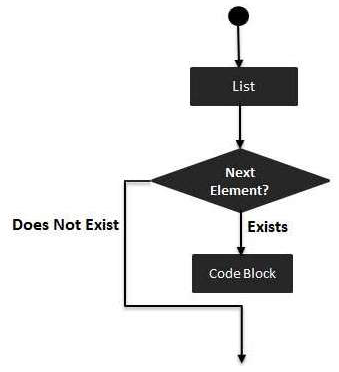# Swift - for-in Loop

The for-in loop iterates over collections of items, such as ranges of numbers, items in an array, or characters in a string −

## Syntax

The syntax of a for-in loop in Swift 4 programming language is −

```for index in var {
statement(s)
}
```Example

```var someInts:[Int] = [10, 20, 30]

for index in someInts {
print( "Value of index is \(index)")
}
```

When the above code is executed, it produces the following result −

```Value of index is 10
Value of index is 20
Value of index is 30
```
swift_loops.htm